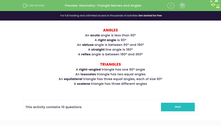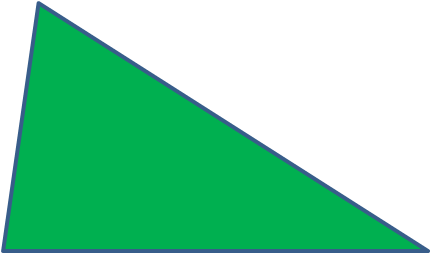# Name Triangles and Angles Correctly

In this worksheet, students will name the different types of angles and triangles.Key stage:  KS 2

Curriculum topic:   Geometry: Properties of Shapes

Curriculum subtopic:   Compare Geometric Shapes

Popular topics:   Geometry worksheets

Difficulty level:#### Worksheet Overview

Here are a few important facts we need to know for our work with triangles!

Angles

right angle is 90º

An acute angle is less than 90ºAn obtuse angle is between 90º and 180ºstraight line angle is 180º

reflex angle is between 180º and 360ºTriangles

A right-angled triangle has one 90º angle.An isosceles triangle has two equal angles.An equilateral triangle has three equal angles, each of size 60º.A scalene triangle has three different angles.That's a lot to remember, but it's all very important stuff to know and will really help you in your geometry work!

If you want to see this introduction again, just click on the red help button that will appear on the screen.

Let's get practising!### What is EdPlace?

We're your National Curriculum aligned online education content provider helping each child succeed in English, maths and science from year 1 to GCSE. With an EdPlace account you’ll be able to track and measure progress, helping each child achieve their best. We build confidence and attainment by personalising each child’s learning at a level that suits them.

Get started